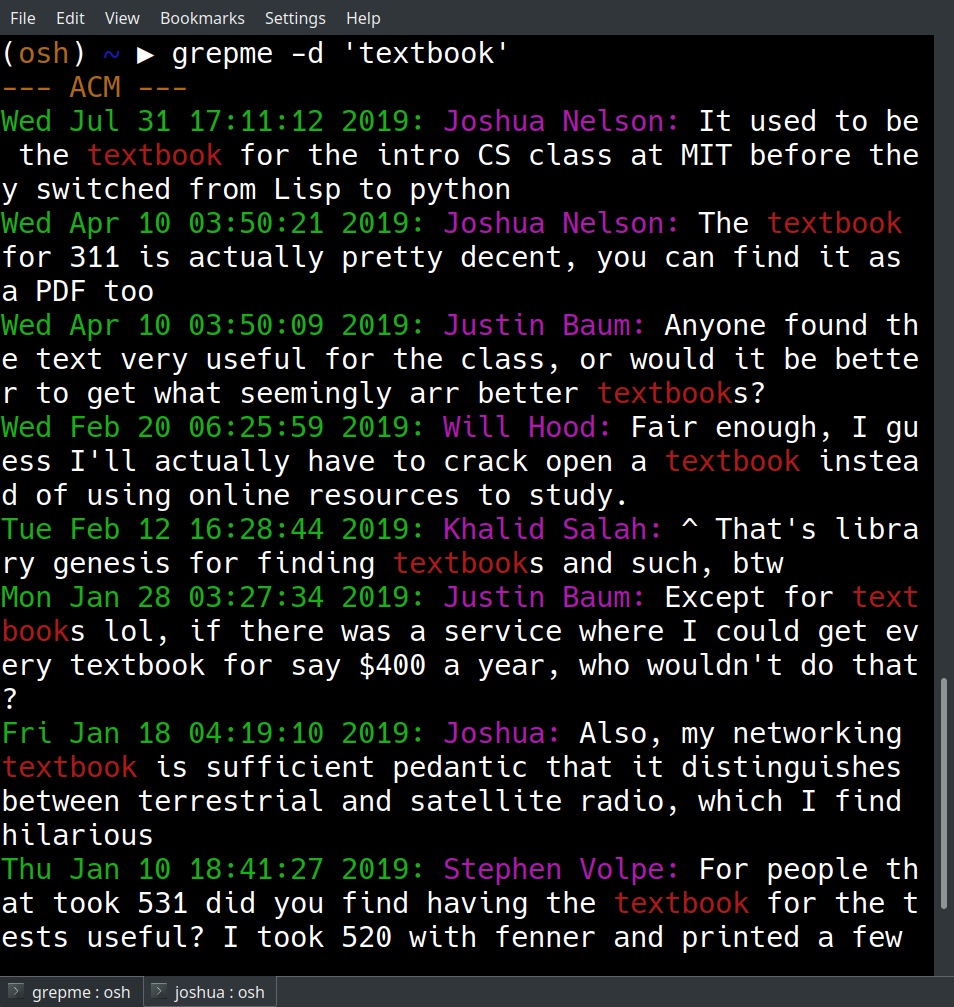# Intro to Python

### A Tour of Python with Real World Code

Created by Joshua Nelson using reveal.js

## Python in 5 minutes

### Declarations

``````
i = 1
d = 1.2
s = "hello, world"
l = [1, 2, 3]
``````
``````
int i = 1;
double d = 1.2;
String s = "hello, world";
int[] i = {1, 2, 3};
``````

### Duck Typing

``````
l = [1, 1.2, "hello, world"]
``````
``````
List<Object> l = Arrays.asList(1, 1.2, "hello, world");
``````

## One slide on dictionaries

What's the difference?

``````
d = {0: 'a', 1: 'b', 2: 'c'}
a = ['a', 'b', 'c']
print(d)  # prints 'a'
print(a)  # prints 'a'
``````

Keys don't have to be integers

``````
d = {'Monday': 'MATH 374', 'Tuesday': 'CSCE 146'}
print(d['Monday'])  # prints 'MATH 374'
``````
Web query parameter example

## What's GrepMe?

GroupMe doesn’t have a working search and it made me sad. So I wrote my own in Python!

Yes, I absolutely used this talk to advertise on of my side projects.## What does it look like?

• Text highlighting
• Searches in reverse chronological order
• Can output dates
• Can search by who liked a message
• Can search multiple groups at a time## How does it work?

Let's look at the source code
Groupme API Reference

## One slide on keyword arguments

What's this do?

``````
def f(**kwargs):
for key, value in kwargs.items():
print(key, value)

f(a='b', c='d')  # prints 'a b\nc d'
``````

## Reference

Compares code in Python to code that does the same thing in Java

1. printing to the console
2. for-each loops
3. generics
5. varargs
6. lambdas

### Printing to the console

``````
print("Hello, world!")
``````
``````
System.out.println("Hello, world!");
``````

### For-Each loops

``````
for i in [1, 2, 3]:
print(i)
``````
``````
for (int i : Arrays.asList(1, 2, 3)) {
System.out.println(i);
}
``````

### Generics

Python doesn't have generics, or rather, everything is generic! It uses duck-typing instead.

``````
def makeList(element):
return [element]
``````
``````
<T> List<T> makeList(T element) {
ArrayList<T> myList = new ArrayList<T>();
return myList;
}
``````

``````
"""
This documents the function 'my_function'

Longer descriptions go here, in plain text
"""
def my_function():
return 4  # guaranteed by IEEE to be random
``````
``````
/**
* This documents the function 'my_function'
*
* Longer descriptions go here, in HTML markup
* @return A random integer between 0 and 1000
*/
int my_function() {
return 4;
}
``````

``````
def print(*args):
for arg in args:
print(arg)
``````
``````
void print(Object ... args) {
for (Object arg : args) {
System.out.println(arg);
}
}
``````

### First-Class and Anonymous Functions

``````
def call_with_one(f):
return f(1)

call_with_one(lambda arg: print(arg))
``````
``````
void call_with_one(IntConsumer f) {
f.accept(1);
}
call_with_one(i -> System.out.println(i));
``````